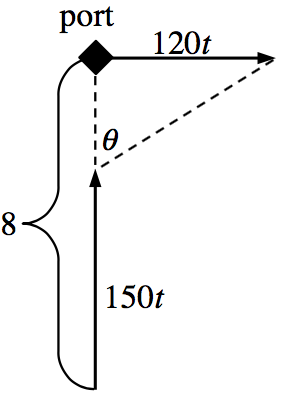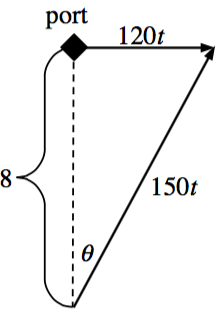### Home > PC3 > Chapter 12 > Lesson 12.1.5 > Problem12-83

12-83.

A boat traveling due north at $150$ km/hr is heading directly for a port. The port is exactly $8$ km away when the captain sees another boat leave the port traveling due east at $120$ km/hr.

1. Determine the angle (from due north) that the captain of the first boat needs to position their telescope to watch the second boat. Express your answer in terms of $t$, the amount of time since the second boat was due north of the first.

Draw and label a diagram. Use consistent units.Write an equation with $θ$ and $t$. Use sine, cosine, or tangent.

2. If the boat that is traveling north wants to catch the boat that is traveling east, what direction will the boat need to head to catch up to the eastbound boat? How long will this take?

Draw a new diagram. The boat traveling due north will now travel at an angle.Write equations with $θ$ and/or $t$. Use sine, cosine, tangent, and/or the Pythagorean Theorem.